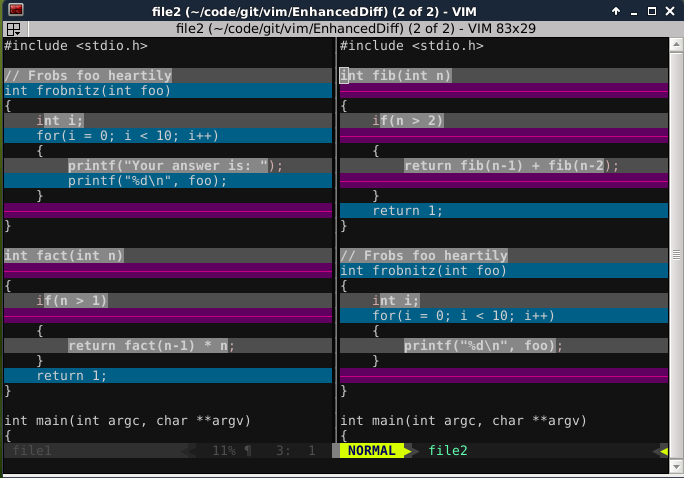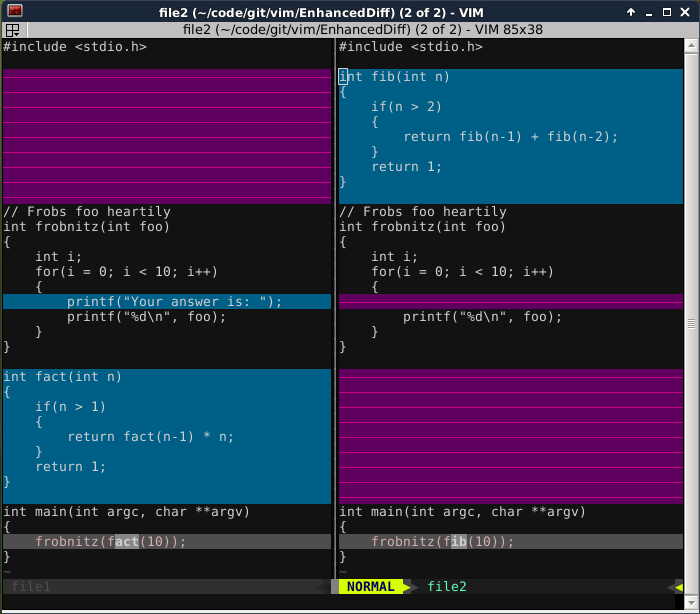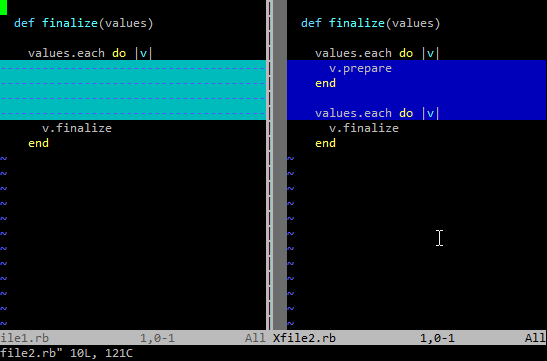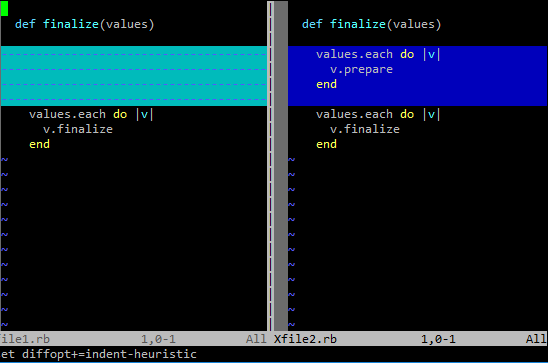## 随 Vim 捆绑 diff 库

```:set diffopt-=internal
```

## 一些例子

### Patience 算法

```#include <stdio.h>

// Frobs foo heartily
int frobnitz(int foo)
{
int i;
for(i = 0; i < 10; i++)
{
printf("%d\n", foo);
}
}

int fact(int n)
{
if(n > 1)
{
return fact(n-1) * n;
}
return 1;
}

int main(int argc, char **argv)
{
frobnitz(fact(10));
}
```

```#include <stdio.h>

int fib(int n)
{
if(n > 2)
{
return fib(n-1) + fib(n-2);
}
return 1;
}

// Frobs foo heartily
int frobnitz(int foo)
{
int i;
for(i = 0; i < 10; i++)
{
printf("%d\n", foo);
}
}

int main(int argc, char **argv)
{
frobnitz(fib(10));
}
```

【译者注】：为方便进行文件差异比较，译者对排版进行了一点小优化。### Indent heuristics 算法

```def finalize(values)

values.each do |v|
v.finalize
end
```

```def finalize(values)

values.each do |v|
v.prepare
end

values.each do |v|
v.finalize
end
```